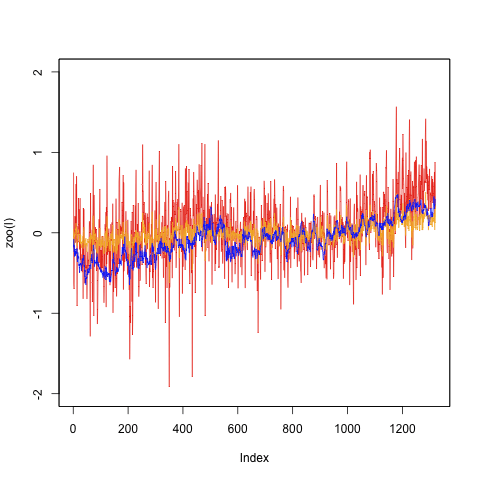Want to share your content on R-bloggers? click here if you have a blog, or here if you don't.

UPDATE:

cce asked for a chart of coastal locations broken out by their SST component and their Land componement.

That’s a little tricky, but once you figure out the mask logic its just addition and such.There are TWO masks: One mask contains ALL zeros, except for coast cells which are fraction of LAND.The other mask contains all zeros except the same coast cell are fraction of water. NOTE. the values here are the contribution of warming from coastal cells. Its SMALL. remember everything is area weighted. But you could look at the trends.

Red is the “land” portion, blue is the sea portion. orange is the sum. index is months from 1900.UPdate: if you want to follow along after this I suggest you get hooked up to raster on R Forge. I’ll be testing out new capability. The code to date uses the CRAN release 1.4.10, but I need to move up to 1.5.2 to test

the new capability. However, baselining the old code probably needs to happen first. A few more bits to test and then we will be shifting to Forge releases of raster ( Cran requires formal testing, so be patient)

```2-Sep-2010,  version 1.5-2

improved speed of gridDistance (JvE)
rasterToPoints can now take a matrix of values and return a brick with layer for each column (suggested by Steven Mosher)
writeRaser (internal saveAs function) takes more care as not to overwrite its own source file, even if overwrite=TRUE```

A weird little benefit of doing things in raster. I can get a look at coastal

if we do

Coast <- Land+SST

the result will be ONLY those areas where they overlap

coast image

Thats because SST has NA where there is 100% Land, and land has NA where there is 100% Ocean

Add those togther and you get NA everywhere BUT the coast

Inv<-getGhcnInventory()

# this line goes away

Inv<-as.GriddedInventory(Inv,Land)

Data<-intersect.InvAnomalies(Inv,Anom)

Anom<-Data\$Anomalies

Inv<-Data\$GridInventory

#####################################################

# this code becomes a one liner with the new ‘pointstoRaster’ basically

# we take the Anomalies which are station based, and aggregate them into a

# cell structure taking the average of all the stations (points) that map to the raster cell.

# see R Forge if you dont understand. stations are points(x,y) you aggregate those points

# into a raster (cells of lat/lon) using a “mean” function. so the work of as.Grid goes away and most

# of the lines that follow. We basically read a V2anomaly structure into a brick. slick.

CellMatrix<-as.GridCells(Anom,Inv)

m<-matrix(nrow=ncell(Land),ncol=ncol(CellMatrix))

b<-brick(Land)

clearValues(b)

m[as.numeric(row.names(CellMatrix)),]<-as.matrix(CellMatrix)

b<-setValues(b,m)

##############################

Weights <- area(b,na.rm=T,weight=T)

Temps<-Weights*b*Land

Weights <- area(SST,na.rm=T,weight=T)